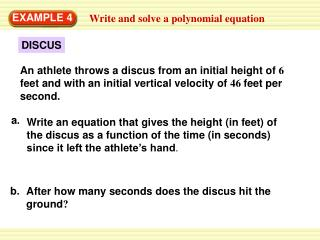Download PresentationEXAMPLE 4

EXAMPLE 4

Download PresentationEXAMPLE 4

- - - - - - - - - - - - - - - - - - - - - - - - - - - E N D - - - - - - - - - - - - - - - - - - - - - - - - - - -
Presentation Transcript

1. a. Write an equation that gives the height (in feet) of the discus as a function of the time (in seconds) since it left the athlete’s hand. b. After how many seconds does the discus hit the ground? EXAMPLE 4 Write and solve a polynomial equation DISCUS An athlete throws a discus from an initial height of 6 feet and with an initial vertical velocity of 46 feet per second.

2. EXAMPLE 4 Write and solve a polynomial equation SOLUTION a. Use the vertical motion model to write an equation for the height h(in feet) of the discus. In this case, v= 46 and s = 6. h =–16t2+vt +s Vertical motion model h =–16t2+46t +6 Substitute 46 for vand 6 for s. b. To find the number of seconds that pass before the discus lands, find the value of tfor which the height of the discus is 0. Substitute 0 for hand solve the equation for t.

3. 1 8 t = – EXAMPLE 4 Write and solve a polynomial equation 0 = –16t2 + 46t + 6 Substitute 0 for h. 0 = –2(8t2 – 23t – 3) Factor out –2. 0 = –2(8t + 1)(t – 3) Factor the trinomial. Find factors of 8 and –3 that produce a middle term with a coefficient of –23. 8t + 1 = 0 or t – 3 = 0 Zero-product property or t = 3 Solve for t.

4. 1 The solutions of the equation are – and 3. A negative solution does not make sense in this situation, so disregard – . 8 1 ANSWER 8 The discus hits the ground after 3 seconds. EXAMPLE 4 Write and solve a polynomial equation

5. ANSWER The discus hits the ground after 2.5 seconds. EXAMPLE 4 Write and solve a polynomial equation 7. WHAT IF?In Example 4, suppose another athlete throws the discus with an initial vertical velocity of 38 feet per second and releases it from a height of 5 feet. After how many seconds does the discus hit the ground?

6. SHOT PUT 8. In a shot put event, an athlete throws the shot put from an initial height of 6 feet and with an initial vertical velocity of 29 feet per second. After how many seconds does the shot put hit the ground? ANSWER The shot put hits the ground after 2 seconds. EXAMPLE 4 Write and solve a polynomial equation